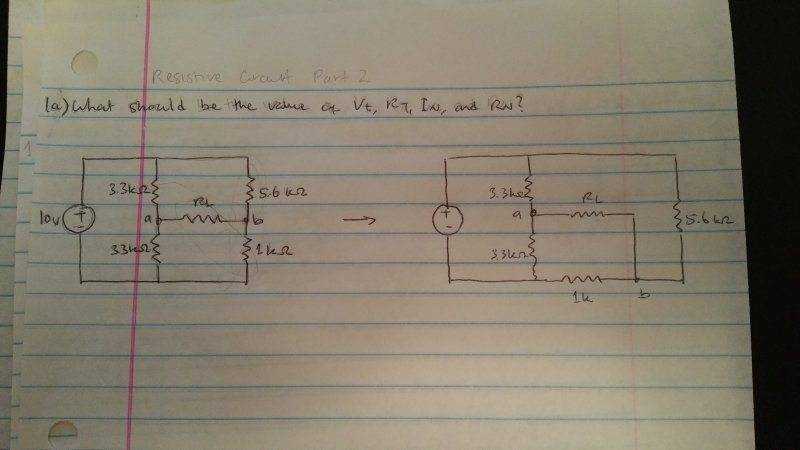# Thevenin and Norton equivalent circuits

Hi guys,
Sorry if all you guys think this question is too easy. But I have a hard time to understand how to group the resistor. I suppose to find the the thevenin and Norton equivalent circuit. This is what i think about the combination of resistor.

Req1= RL+1k
Req2= Req1 ll 3.3k (3.3k after node a)
Req3= Req2 + 3.3k (3.3k before node a)
Req4= Req3 ll 5.6k

Is my prediction correct?phinds
Gold Member
2021 Award
I have no idea what you mean. I always find it best to redraw the circuit with the load nodes A and B shown on the right, one above the other, with the rest of the circuit redrawn as simply as possible to accommodate that and THEN worry about series/parallel reductions.

NascentOxygen
Staff Emeritus
When you redraw the circuit, omit the load. The load is not part of the Thèvenin equivalent of the source.

You might understand things better if you first work out the Thèvenin voltage, and leave the resistance calculation until last.First of all I have to apologize for my mistake!
I thought it was a very simple question and
it was only an example how to proceed generally.
I think it was better to recommend a link how to solve this kind of problems. There are a lot of links on the web treating specifically this.

For instance: http://www.calvin.edu/~svleest/circuitExamples/TheveninNorton/

NascentOxygen
Staff Emeritus
As a check, you could imagine a short-circuit wire between a and b, and calculate how much current would flow. Does your Thévenin equivalent suggest that same value?

Last edited:
phinds
Gold Member
2021 AwardFirst of all I have to apologize for my mistake!
I thought it was a very simple question and
it was only an example how to proceed generally.
I think it was better to recommend a link how to solve this kind of problems. There are a lot of links on the web treating specifically this.

For instance: http://www.calvin.edu/~svleest/circuitExamples/TheveninNorton/
Yes, and people with initiative will generally look for such solutions online but some just come here, which is fine, and we try to help them HERE rather than just doing the online search that they could have done for themselves.

Thank you NascentOxygen .The short-circuit current according to Thevenin rule V=I*Rth+Vth

If V=0 then Isc=-Vth/Rth Isc=-3.485/2.4985=-1.39484 mA.

If we shall short-circuit point a and b the total current will be 10/(3.3*5.6/(3.3+5.6)+3.3*1/(3.3+1))=3.51636 mA.

The current flowing in each resistance will be:

for 3.3k 3.51636*5.6/(3.3+5.6)=2.2125 mA.

for 5.6k 1.3038 mA.

for second 3.3k -0.81776 [it leaves the point a].

and for 1k -2.6986mA[it leaves the point b].

The sum of currents in point a will be:

Isca+2.2125-0.81776=0 Isca= -1.3948 mA.

-Iscb+1.3038-2.6986=0 Iscb=-1.3948